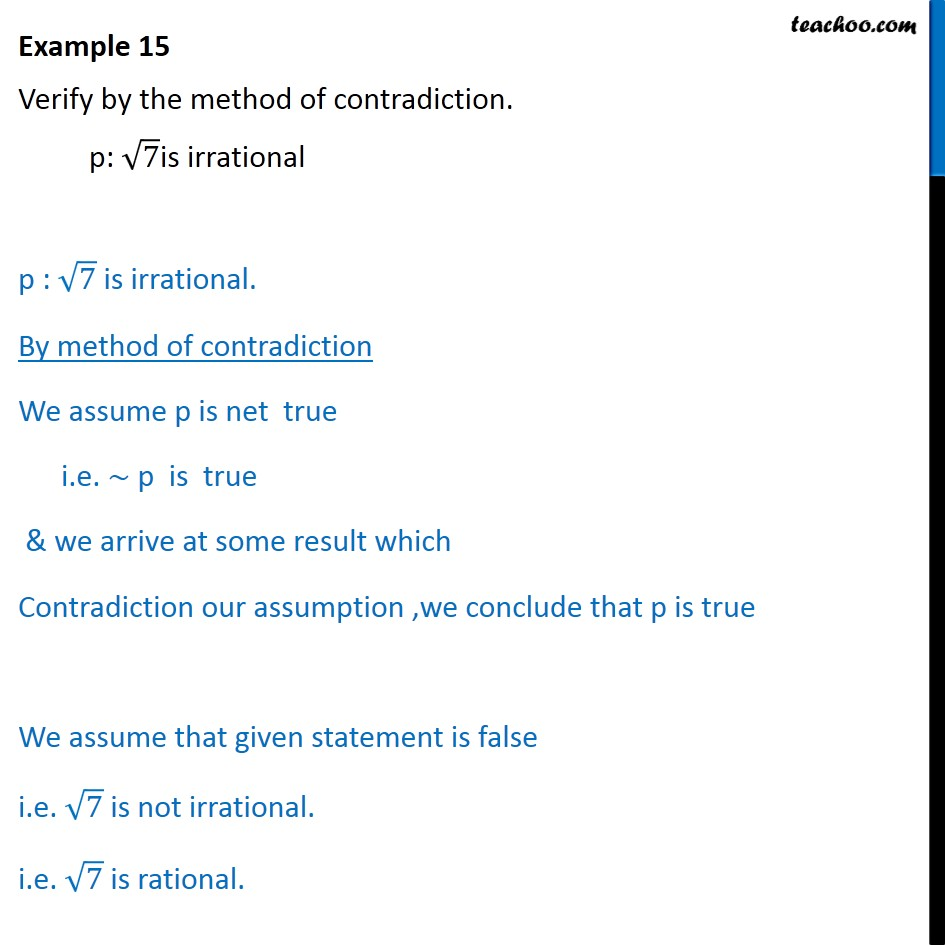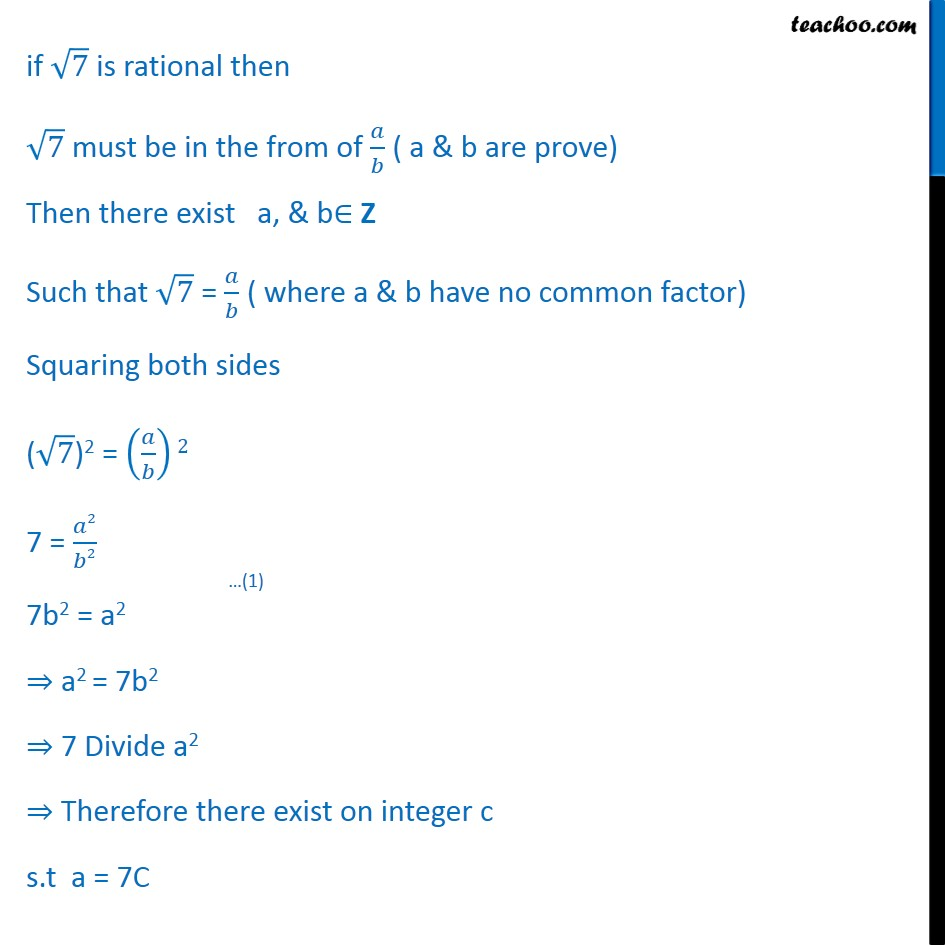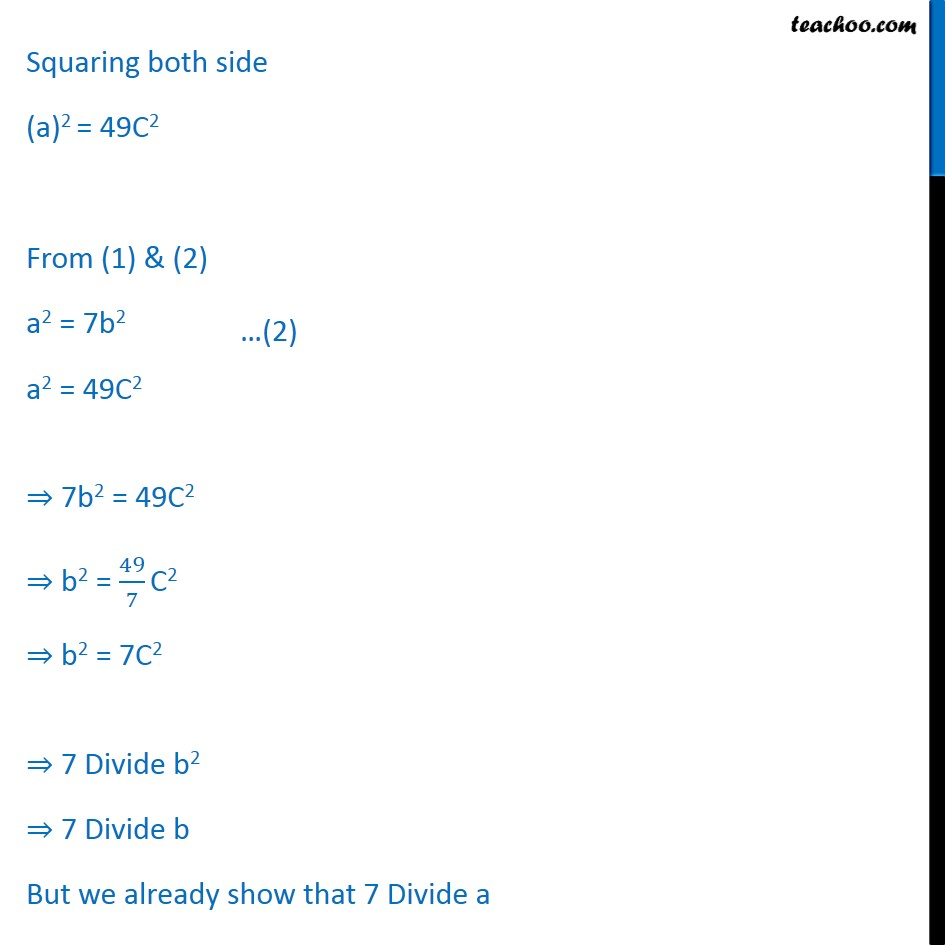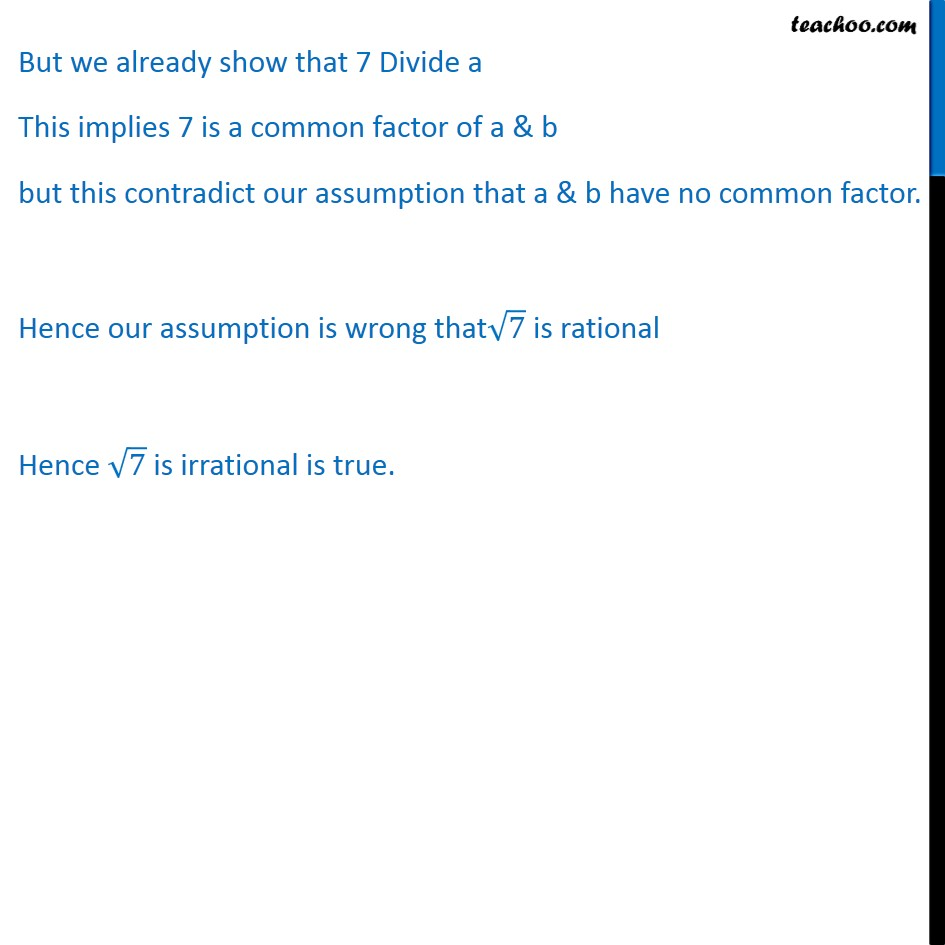Subscribe to our Youtube Channel - https://you.tube/teachoo

1. Chapter 14 Class 11 Mathematical Reasoning
2. Concept wise
3. Proving True - By Contradiction

Transcript

Example 15 Verify by the method of contradiction. p: ﷐﷮7﷯is irrational p : ﷐﷮7﷯ is irrational. By method of contradiction We assume p is net true i.e. ∼ p is true & we arrive at some result which Contradiction our assumption ,we conclude that p is true We assume that given statement is false i.e. ﷐﷮7﷯ is not irrational. i.e. ﷐﷮7﷯ is rational. if ﷐﷮7﷯ is rational then ﷐﷮7﷯ must be in the from of ﷐𝑎﷮𝑏﷯ ( a & b are prove) Then there exist a, & b∈ Z Such that ﷐﷮7﷯ = ﷐𝑎﷮𝑏﷯ ( where a & b have no common factor) Squaring both sides (﷐﷮7﷯)2 = ﷐﷐𝑎﷮𝑏﷯﷯2 7 = ﷐𝑎2﷮𝑏2﷯ 7b2 = a2 ⇒ a2 = 7b2 ⇒ 7 Divide a2 ⇒ Therefore there exist on integer c s.t a = 7C Squaring both side (a)2 = 49C2 From (1) & (2) a2 = 7b2 a2 = 49C2 ⇒ 7b2 = 49C2 ⇒ b2 = ﷐49﷮7﷯ C2 ⇒ b2 = 7C2 ⇒ 7 Divide b2 ⇒ 7 Divide b But we already show that 7 Divide a But we already show that 7 Divide a This implies 7 is a common factor of a & b but this contradict our assumption that a & b have no common factor. Hence our assumption is wrong that﷐﷮7﷯ is rational Hence ﷐﷮7﷯ is irrational is true.